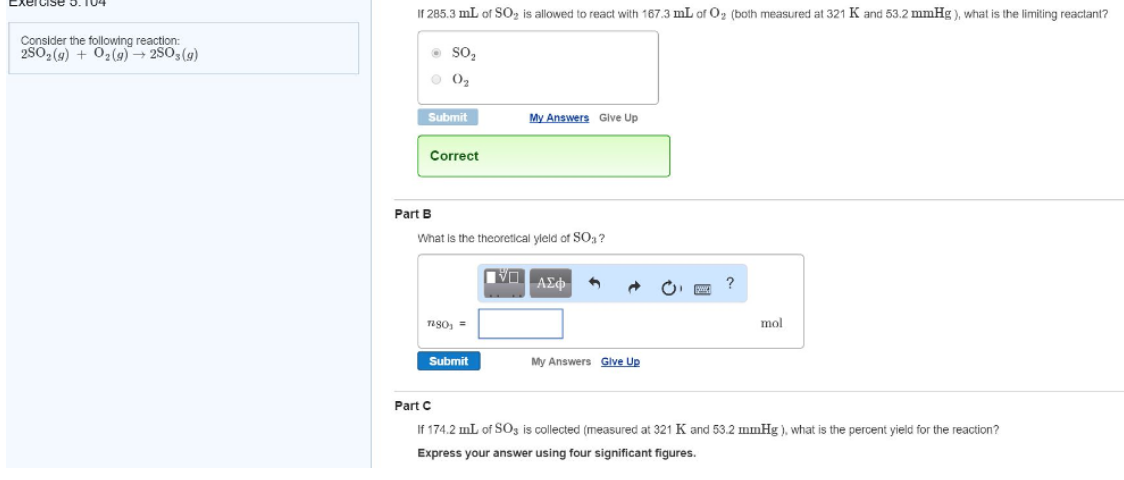# Problem: Consider the following reaction:2SO2(g) + O2(g) → 2SO3(g)If 285.3 mL of SO2 is allowed to react with 167.3 mL of O2 (both measured al 321 K and 53.2 mmHg), what is the limiting reactant?What is the theoretical yield of SO3?If 174.2 mL of SO3 is collected (measured at 321 K and 53.2 mmHg), what is the percent yield for the reaction?

###### FREE Expert Solution
82% (326 ratings)###### Problem Details

Consider the following reaction:

2SO2(g) + O2(g) → 2SO3(g)

If 285.3 mL of SO2 is allowed to react with 167.3 mL of O2 (both measured al 321 K and 53.2 mmHg), what is the limiting reactant?

What is the theoretical yield of SO3?

If 174.2 mL of SO3 is collected (measured at 321 K and 53.2 mmHg), what is the percent yield for the reaction?